# Electro-Thermo-Mechanical Modeling of a V-beam Actuator

HOME / Applications / Electro-Thermo-Mechanical Modeling of a V-beam Actuator

### Applications

Used Tools:## Introduction

Micro-electro-mechanical system (MEMS) devices can be categorized, based on the actuation source, into four main types:  electrostatic, electromagnetic, piezoelectric and electro-thermal.

Electro-thermal actuation is based on thermal expansion caused by joule heating, which can be achieved by passing current through the beams of the actuator to cause resistive heating.

Electro thermal actuators are useful in many applications that require converting electrical input into mechanical output, such as tunable parallel plate capacitors, optical modulators, RF MEMS switches and micro-manipulators. These actuators are characterized by compactness, stability and high force generated under low actuation voltage.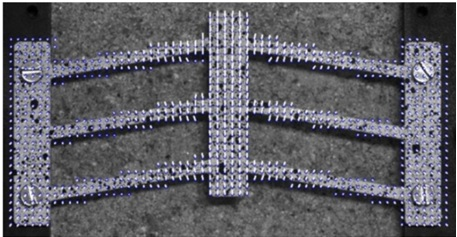Figure 1 - Electrothermal actuator .

## Problem description

A Magnetostatic simulation coupled to thermo-structural analysis is performed to investigate the thermal and mechanical behavior of the V-beam electro-thermal actuator. The studied model consists of amm shuttle attached to 3 pairs of beams with 26.4 mm length and 1 mm width for each beam. The beams are attached to 2 anchors of 6 mm width and 30 mm length each.

The thickness of the whole thermal actuator along Z axis is 1 mm.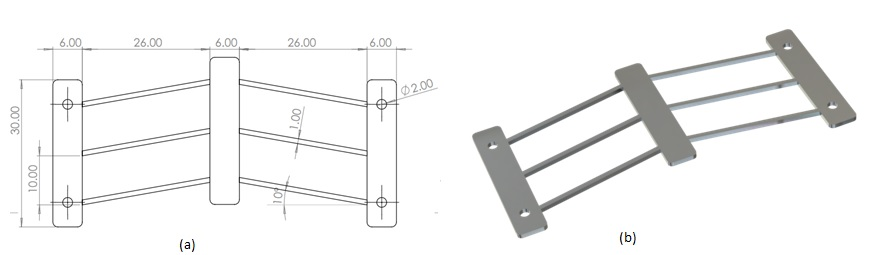Figure 2 - a) Drawing of the V-beam thermal actuator   b) 3D Model of the thermal actuator.

## Simulation Setup

The Magnetostatic module of EMS, coupled to steady-state thermal and structural analysis, is used to compute and visualize the temperature distribution and the displacement of the shuttle.

To perform the analysis using EMS, first, the appropriate material is selected for all solids; second, the necessary electromagnetic inputs are defined; third, the necessary thermal inputs are applied; forth, the structural boundary conditions are applied and finally, the entire model is meshed, and the solver is launched.

### Materials

In our case study, the following properties of material are used (Table1):

Table 1 - Material properties.

 Part Density (Kg/) Relative permeability Electrical conductivity (S/m) Specific heat capacity (J/kg. K) Thermal conductivity (W/m. K) Elastic Modulus (Pa) Poisson’s ratio Thermal expansion coefficient (1/K) Aluminum 2700 1 3.57 E+07 910 235 71  E+09 0.36 23.1 E-06 air 0 1 0 1000 0.024 Not required

### Electromagnetic Inputs:

The thermal actuator is defined as a solid coil carrying a 40 A DC current.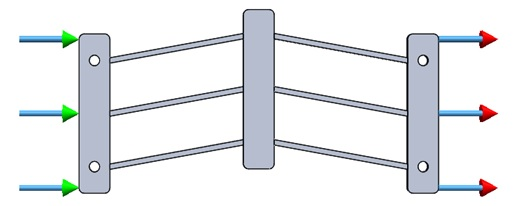Figure 3 - Applied current input .

### Thermal Input:

The thermal convection for the ambient air is specified as follows:

1. The initial (ambient) temperature of the simulation is set to 303.15 K .
2. Convection coefficient on the surrounding air is set to 15 W/m2K .

### Mechanical boundary conditions:

Fixed boundary conditions are applied to both anchors of the thermal actuator, as shown in the figure 4: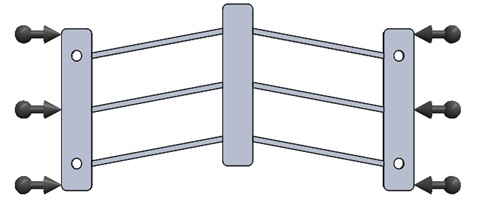Figure 4 - Applied mechanical boundary conditions.

## Meshing:

A Mesh Control is applied to the shuttle and the 6 beams where the maximum temperature and displacement is expected, as shown in figure5.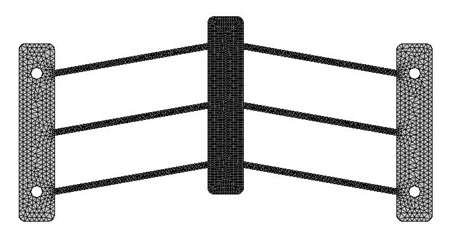Figure 5 - Mesh of the model.

## Results

EMS offers the possibility to visualize results as 3D plots and curves, these results include: applied current density, temperatureand heat flux distribution, mechanical displacement, stress, etc.

The Table 2 illustrates the DC resistance of the simulated V-beam thermal actuator, as computed by EMS compared with the analytical result.

Table 2 - Resistance of the thermal actuator compared with the analytical value.

 EMS Analytical ( reference) Electric Resistance () 5.59 E-04 5.73 E-04I=40 A (current intensity)

Ra =4.96E-04(Ambient temperature resistance)

Ac =1553.6 m(convection area)

hc=15 W/.K(convective heat transfer coefficient)

k=3.9.10-3 1/°C (temperature coefficient)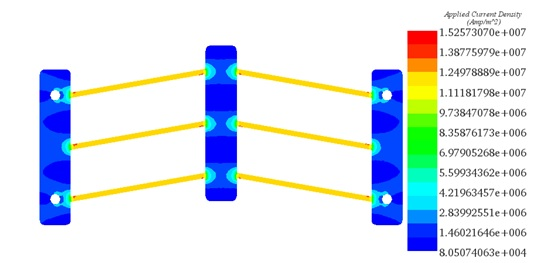Figure 6 - Plot of the applied current density.

The Figure 6 shows the current density distribution in the V-beam thermal actuator.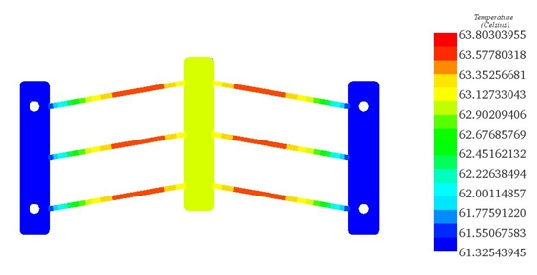Figure 7 - Plot of temperature distribution .

The Figure 7 shows the temperature distribution in the V-beam thermal actuator that achieves a maximum value of 63.8 °C.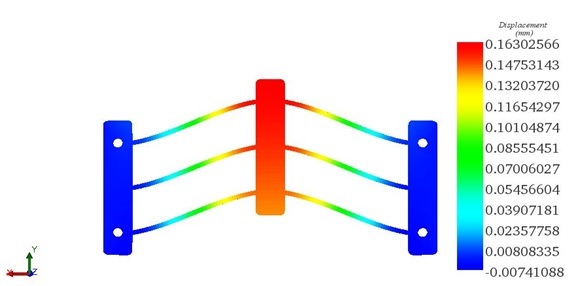Figure 8 - plot of Y displacement plot .

The Figure 8 shows the displacement distribution along Y axis in the V-beam thermal actuator that achieves a maximum value of 0.163 mm.

The maximum temperature of 63.8 °C is located on the beams due to the current concentration in this location. For the displacement, the shuttle experiences the maximum mechanical deflection of 0.163 mm along Y axis.

Table 3 shows the correlation of the temperature and the displacement between EMS and the reference.

Table 3: Comparative table between EMS and the reference .

 EMS Reference  Temperature (°C) 63.8 63.5 Displacement (mm) 0.163 0.165

## Conclusion

This simulation provided an accurate investigation of the thermal and mechanical response of the actuator when passing electric current through it. In fact, the micro-actuators are devices that convert energy into action capable of producing micro motion. The micro-motion is used to position elements in MEMS.

The numerical simulation results, achieved through EMS Simulation Software, of the behavior of the V-beam electro-thermal actuator, were found in good agreement with the reference .

## References

. Radu-Stefan Chiorean, “Electro-Thermo-Mechanical Modeling of V-beam Actuator”,8th international conference interdisciplinarity in engineering INTER-ENG 2014,9-10 october 2014, Tirgu-Mures, Romania.